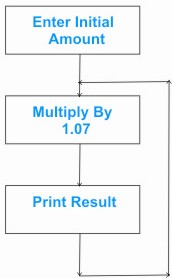HOME MATH DICTIONARY DOWNLOAD FEEDBACK DISCLAIMER
 Question: What is an Iterative Procedure ? Answer: In everyday language the word reiterate means to say again, to repeat. In mathematics an iterative procedure is a repetitive process. Here is an example, A calculator can be used to find how a sum of money, \$1000 which grows when the interest rate is 7% per year by entering 1000 and then repeatedly multiplying by 1.07.Amount at the end of year 1: \$1000 x 1.07 = \$1070 Amount at the end of year 2: \$1070 x 1.07 = \$1144.90 Amount at the end of year 3: \$1144.90 x 1.07 = \$1225.043 The amount \$an at the end of the year n and the amount \$an + 1 at the end of year n + 1 are connected by the iterative relation a n + 1 = 1.07an